## Diophantine Equation--Linear

A linear Diophantine equation (in two variables) is an equation of the general form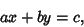(1)

where solutions are sought with,, and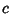Integers. Such equations can be solved completely, and the first known solution was constructed by Brahmagupta. Consider the equation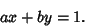(2)

Now use a variation of the Euclidean Algorithm, lettingand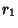(3)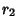(4)(5)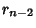(6)

Starting from the bottom gives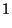(7)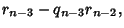(8)

so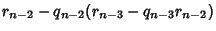(9)

Continue this procedure all the way back to the top.

Take as an example the equation(10)

Proceed as follows.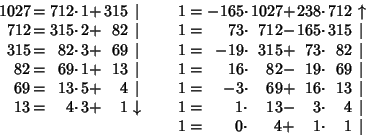The solution is therefore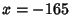,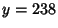. The above procedure can be simplified by noting that the two left-most columns are offset by one entry and alternate signs, as they must since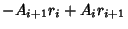(11)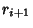(12)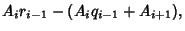(13)

so the Coefficients of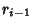andare the same and(14)

Repeating the above example using this information therefore givesand we recover the above solution.

Call the solutions to(15)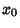and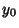. If the signs in front oforare Negative, then solve the above equation and take the signs of the solutions from the following table:

 equation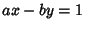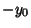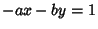In fact, the solution to the equation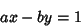(16)

is equivalent to finding the Continued Fraction for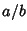, withandRelatively Prime (Olds 1963). If there areterms in the fraction, take the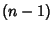th convergent. But(17)

so one solution is,, with a general solution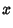(18)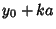(19)

withan arbitrary Integer. The solution in terms of smallest Positive Integers is given by choosing an appropriate.

Now consider the general first-order equation of the form(20)

The Greatest Common Divisor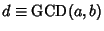can be divided through yielding(21)

where,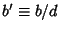, and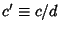. If, thenis not an Integer and the equation cannot have a solution in Integers. A necessary and sufficient condition for the general first-order equation to have solutions in Integers is therefore that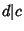. If this is the case, then solve(22)

and multiply the solutions by, since(23)

References

Courant, R. and Robbins, H. Continued Fractions. Diophantine Equations.'' §2.4 in Supplement to Ch. 1 in What is Mathematics?: An Elementary Approach to Ideas and Methods, 2nd ed. Oxford, England: Oxford University Press, pp. 49-51, 1996.

Dickson, L. E. Linear Diophantine Equations and Congruences.'' Ch. 2 in History of the Theory of Numbers, Vol. 2: Diophantine Analysis. New York: Chelsea, pp. 41-99, 1952.

Olds, C. D. Ch. 2 in Continued Fractions. New York: Random House, 1963.